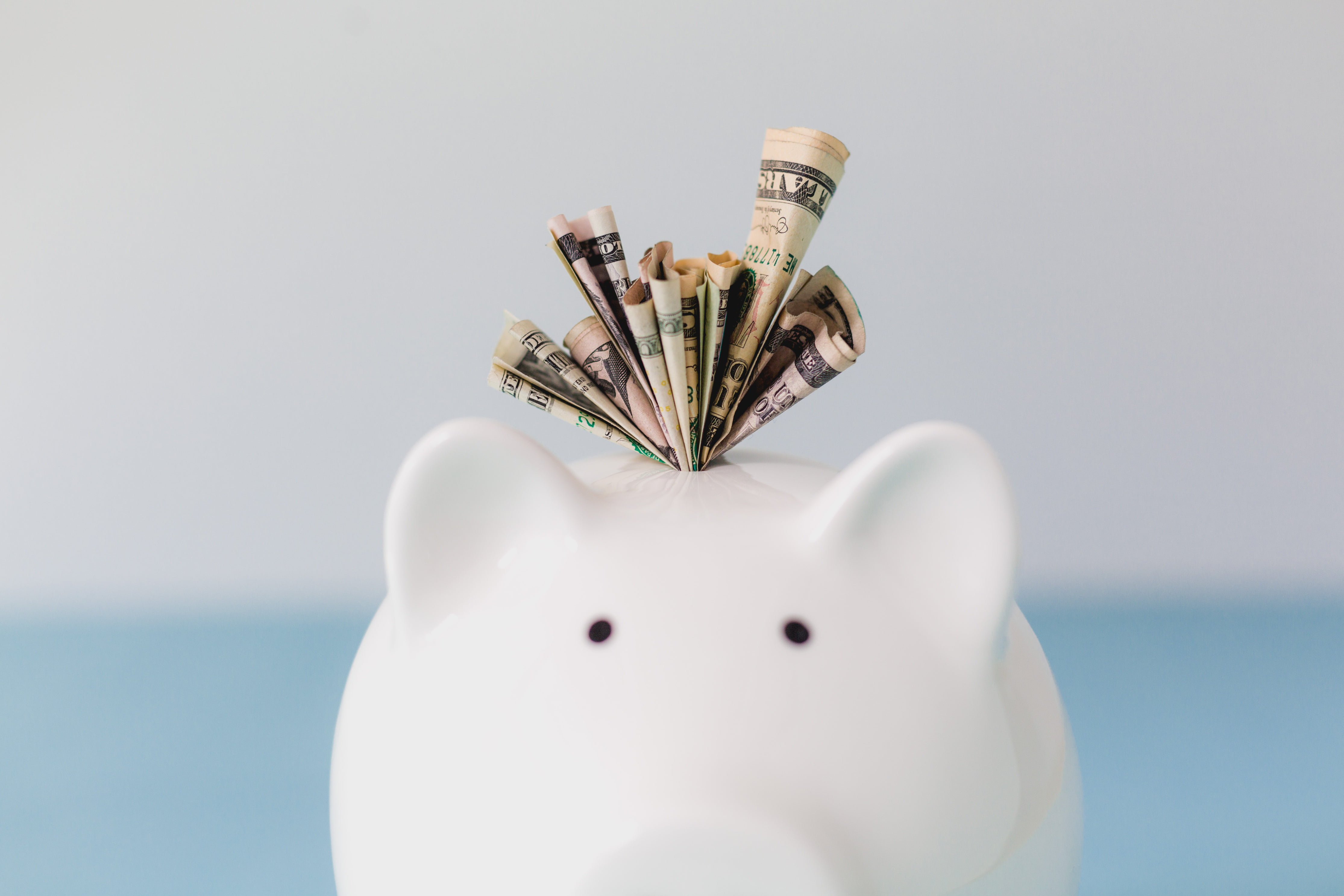<- Back

# ROI: Return on investment

ROI (Return on Investment) refers to the level of returns made through an investment by measuring net income against its original cost over a period of time.## What is ROI?

It’s a popular method to measure the profitability and efficiency of an economic entity. When it comes to your own personal investments, it serves as a useful indicator to compare and rank different investments within a portfolio. The logic follows that the higher the ROI, the more profitable (and therefore effective) an investment is. So clearly, we want our investments to have a high ROI!

KEY TAKEAWAYS

• ROI (Return on Investment) is a useful measure of profitability
• It enables investors to assess the level of returns made through an investment by measuring net income against its original cost over a period of time
• The higher the ROI, the more profitable an investment is
• Anywhere from 7 to 10% is usually considered a good ROI for long-term investors## How to calculate ROI?

To calculate ROI, the net profit of an investment is divided by the initial cost of the investment, which is then expressed as a percentage or a ratio.

## Return on investment formula

ROI = ( Current Value of Investment - Cost of Investment ) / ( Cost of Investment )

Return on Investment Examples:

If you bought a house for \$700,000 and proceeded to sell it for \$900,000, you gain a profit of \$200,000 (net profit). If you then divide the net profit (\$200,000) by the cost of your total initial investment (\$700,000) and then multiply by 100, your total ROI percentage ends up being 28.5%.

Similarly, if you invested \$5,000 into Apple stocks and after 12 months find they’re up to \$5,500, you have earned a net profit of \$500. If you divide the net profit (\$500) by the cost of your investment (\$5,000) and multiply by 100, your total ROI percentage ends up being 10%.

## What is a good ROI?

When it comes to your own stocks, anywhere from 7 to 10% is usually considered a good ROI for long-term investors. However much we would like a 20%, 30% or even 40% return, it’s pretty significant in the world of investing. If you have a high risk appetite and are comfortable in trading in volatile investments, then something higher than 10% is possible. But it’s important to know your limits and to be realistic!

We are committed to educating and empowering women to take control of their finances and to live life on their own terms.

CONTACT